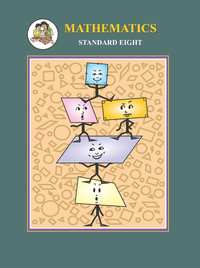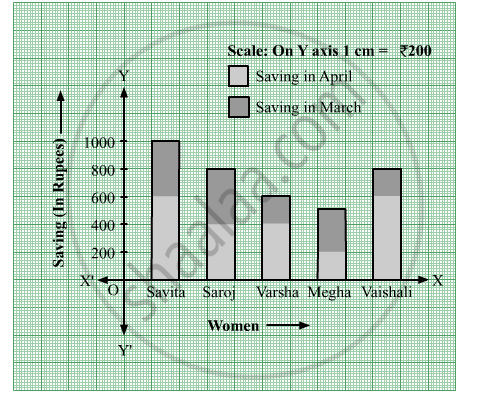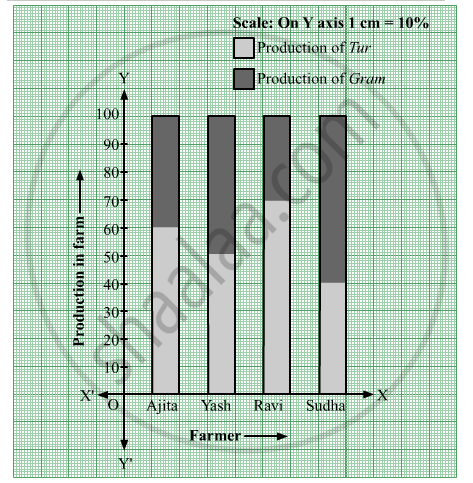# Balbharati solutions for Mathematics 8th Standard Maharashtra State Board chapter 11 - Statistics [Latest edition]

#### Chapters## Chapter 11: Statistics

Practice Set 11.1Practice Set 11.2Practice Set 11.3
Practice Set 11.1 [Pages 69 - 70]

### Balbharati solutions for Mathematics 8th Standard Maharashtra State Board Chapter 11 Statistics Practice Set 11.1 [Pages 69 - 70]

Practice Set 11.1 | Q 1 | Page 69

The following table shows the number of saplings planted by 30 students. Fill in the boxes and find the average number of saplings planted by each student.

 No. of saplings(Scores) xi No. of students(frequncy) fi fi x xi 1 4 4 2 6 _______ 3 12 _______ 4 8 _______ N = _________ ∑fixi =  ______

Mean bar "x" = square/"N"

= square/square
= square
∴ The average number of trees planted square

Practice Set 11.1 | Q 2 | Page 70

The following table shows the electricity (in units) used by 25 families of Eklara village in a month of May. Complete the table and answer the following questions.

 Electricity used(units) x_i No. of Families(frequency) f_i f_ix_i 30 7 _________ 45 2 __________ 60 8 __________ 75 5 __________ 90 3 __________ N = _________ sum f_ix_i =______

(1) How many families use 45 units electricity?
(2) State the score, the frequency of which is 5.
(3) Find N, and sum f_ix_i
(4) Find the mean of electricity used by each family in the month of May.

Practice Set 11.1 | Q 3 | Page 70

The number of members in the 40 families in Bhilar are as follows:
1, 6, 5, 4, 3, 2, 7, 2, 3, 4, 5, 6, 4, 6, 2, 3, 2, 1, 4, 5, 6, 7, 3, 4, 5, 2, 4, 3, 2, 3, 5, 5, 4, 6, 2, 3, 5, 6, 4, 2. Prepare a frequency table and find the mean of members of 40 families.

Practice Set 11.1 | Q 4 | Page 70

The number of Science and Mathematics projects submitted by Model high school, Nandpur in last 20 years at the state level science exibition is:
2 3 4 1 2 3 1 5 4 2 3 1 3 5 4 3 2 2 3 2. Prepare a frequency table and find the mean of the data.

Practice Set 11.2 [Pages 71 - 72]

### Balbharati solutions for Mathematics 8th Standard Maharashtra State Board Chapter 11 Statistics Practice Set 11.2 [Pages 71 - 72]

Practice Set 11.2 | Q 1 | Page 71

Observe the following graph and answer the question.(1) State the type of the graph.
(2) How much is the savings of Vaishali in the month of April?
(3) How much is the total savings of Saroj in the months March and April?
(4) How much more is the total savings of Savita than the total savings of Megha?
(5) Whose savings in the month of April is the least?

Practice Set 11.2 | Q 2 | Page 72

The number of boys and girls, in std 5 to std 8 in a Z.P. school is given in the table. Draw a subdivided bar graph to show the data.
(Scale : On Y axis, 1 cm = 10 students)

 Standard 5th 6th 7th 8th Boys 34 26 21 25 Girls 17 14 14 20
Practice Set 11.2 | Q 3 | Page 72

In the following table number of trees planted in the year 2016 and 2017 in four towns is given. Show the data with the help of subdivided bar graph.

 Town → Karjat Wadgoan Shivapur Khandala Year ↴ 2016 150 250 200 100 2017 200 300 250 150
Practice Set 11.2 | Q 4 | Page 72

In the following table, data of the transport means used by students in 8th standard for commutation between home and school is given. Draw a subdivided bar diagram to show the data.
(Scale : On Y axis : 1 cm = 500 students)

 Town → Paithan Yeola Shahapur Mean of Communication  ↴ cycle 3250 1500 1250 Bus and Auto 750 500 500 On foot 1000 1000 500
Practice Set 11.3 [Pages 73 - 74]

### Balbharati solutions for Mathematics 8th Standard Maharashtra State Board Chapter 11 Statistics Practice Set 11.3 [Pages 73 - 74]

Practice Set 11.3 | Q 1 | Page 73

Show the following information by percentage bar graph.

 Division of standard 8 A B C D Number of students securring grade A 45 33 10 15 Total number of students 60 55 40 75
Practice Set 11.3 | Q 2 | Page 74

Observe the following graph and answer the questions.(1) State the type of the bar graph.
(2) How much percent is the Tur production to total production in Ajita'a farm?
(3) Compare the production of Gram in the farms of Yash and Ravi and state whose percentage of production is more and by how much?
(4) Whose percentage production fo Tur is the least?
(5) State production percentages of Tur and gram in Sudha's farm

Practice Set 11.3 | Q 3 | Page 74

The following data is collected in a survey of some students of 10th  standard from some schools. Draw the percentage bar graph of the data.

 School 1st 2nd 3rd 4th Inclination towards science stream 90 60 25 16 Inclination towards commerce stream 60 20 25 24

## Chapter 11: Statistics

Practice Set 11.1Practice Set 11.2Practice Set 11.3## Balbharati solutions for Mathematics 8th Standard Maharashtra State Board chapter 11 - Statistics

Balbharati solutions for Mathematics 8th Standard Maharashtra State Board chapter 11 (Statistics) include all questions with solution and detail explanation. This will clear students doubts about any question and improve application skills while preparing for board exams. The detailed, step-by-step solutions will help you understand the concepts better and clear your confusions, if any. Shaalaa.com has the Maharashtra State Board Mathematics 8th Standard Maharashtra State Board solutions in a manner that help students grasp basic concepts better and faster.

Further, we at Shaalaa.com provide such solutions so that students can prepare for written exams. Balbharati textbook solutions can be a core help for self-study and acts as a perfect self-help guidance for students.

Concepts covered in Mathematics 8th Standard Maharashtra State Board chapter 11 Statistics are Arithmetic Mean - Raw Data, Subdivided Bar Graph/Diagram, Percentage Bar Graph/Diagram.

Using Balbharati 8th Standard solutions Statistics exercise by students are an easy way to prepare for the exams, as they involve solutions arranged chapter-wise also page wise. The questions involved in Balbharati Solutions are important questions that can be asked in the final exam. Maximum students of Maharashtra State Board 8th Standard prefer Balbharati Textbook Solutions to score more in exam.

Get the free view of chapter 11 Statistics 8th Standard extra questions for Mathematics 8th Standard Maharashtra State Board and can use Shaalaa.com to keep it handy for your exam preparation